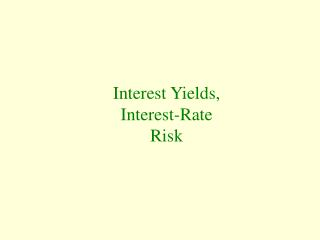DownloadDownload PresentationInterest Yields, Interest-Rate Risk

# Interest Yields, Interest-Rate Risk

Download Presentation## Interest Yields, Interest-Rate Risk

- - - - - - - - - - - - - - - - - - - - - - - - - - - E N D - - - - - - - - - - - - - - - - - - - - - - - - - - -
##### Presentation Transcript

1. Interest Yields, Interest-Rate Risk

2. Interest Rates • To understand the risks owing to interest rate variations, how to minimize those risks, or how to profit from them requires an understanding of how interest rates and the prices of financial instruments are related. • Principal is the amount of credit extended when one makes a loan or purchases a bond.

3. Interest Yields • Interest is the payment by the issuer of a financial instrument that compensates the purchaser for the use of their funds. • The interest rate is the amount of interest expressed as a percentage of the principal. • Capital gain is a rise in the value of a financial instrument at the time it is sold relative to its market value at the time it was purchased.

4. Discounted Present Value • Discounted present value is the value today of a payment to be received at a future date. • Calculating discounted present value: • The value today of a payment to be received at a future date. • Payment one year from now/(1+ r). • Discounted present value of payment to be received n years in the future: • Payment n years from now/(1+ r)n.

5. Present Values of a Future Dollar Compounded Annual Interest RateYear 3% 5% 8% 10% 20%1 .971 .952 .926 .909 .833 2 .943 .907 .857 .826 .694 3 .915 .864 .794 .751 .578 4 .889 .823 .735 .683 .482 5 .863 .784 .681 .620 .402 6 .838 .746 .630 .564 .335 7 .813 .711 .583 .513 .279 8 .789 .677 .540 .466 .233 9 .766 .645 .500 .424 .194 10 .744 .614 .463 .385 .162

6. Calculating the Yield to Maturity • Perpetuity: • A bond with an infinite term to maturity. • Perpetuity price = C/r. • Simple rule: • Prices of existing bonds are inversely related to changing market interest rates.

7. Term to Maturity and Interest-Rate Risk • Interest rate risk is the possibility that the market value of a financial instrument will change as interest rates vary. • Capital loss is a decline in the market value of a financial instrument at the time it is sold as compared with its market value at the time it was purchased.

8. The Term Structure • Term Structure of interest rates is the relationship among yields on financial instruments with identical risk, liquidity, and tax characteristics but differing terms to maturity. • Yield Curve is a chart illustrating the relationship among yields on bonds that differ only in their term to maturity.

9. Yield Curves Typically, yield curves slope upward: interest yields rise at longer terms to maturity.

10. Yield Curves • Economists offer three fundamental explanations for why yield curves are typically upward sloping. • Segmented Markets • Expectations Theory • Preferred Habitat Theory

11. Segmented Markets Theory • Segmented markets theory is a theory of the term structure of interest rates that views bonds with differing maturities as nonsubstitutable, so their yields differ because they are determined in separate markets. • Drawbacks to theory: • Yields tend to move together. • Does not explain natural tendency of the yield curve to slope upward or downward.

12. Expectations Theory • Expectations theory explains how expectations about future yields can cause yields on instruments with different maturities to move together. • It can provide insight into why the yield curve may systematically slope upward or downward: • An upward-sloping yield curve indicates a general expectation by savers that short-term interest rates will rise. • A downward-sloping yield curve indicates a general expectation that short-term interest rates will decline.

13. The Preferred Habitat Theory • Preferred habitat theory is a theory of the term structure of interest rates that views bonds as imperfectly substitutable, so yields on longer-term bonds must be greater than those on shorter-term bonds even if short-term interest rates are not expected to rise or fall. • Term premium is the amount by which the yield on a long-term bond must exceed the yield on a short-term bond to make individuals willing to hold either bond if they expect short-term bond yields to remain unchanged.

14. The Risk Structure of Interest Rates • Risk structure of interest rates is the relationship among yields on financial instruments that have the same maturity but differ because of variations in default risk, liquidity, and tax rates. • Default risk is the chance that an individual or a firm that issues a financial instrument may be unable to honor its obligations to repay the principal and/or to make interest payments.